Next: Projection Operators for Energy Up: Plane-Wave Solutions Previous: Plane-Wave Solutions

## Spin

We have just seen how one can create a state of arbitrary momentum by Lorentz transforming the solution for a particle at rest. In a similar manner, we can create a state of arbitrary spin polarized along the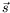direction by applying the rotation operator,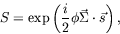(5.198)

to the solution for a particle at rest and polarized in the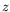direction. The defining relationship for such a state is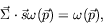(5.199)

where the spinor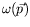corresponds to a particle polarized along the direction of the unit vector. Remember that the spin operator is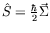and thus the eigenvalue of the spin operator in this case is really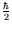, as we might expect.

Let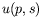denote a spinor of positive energy, momentum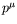, and spin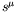. The spin vector is defined as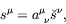(5.200)

where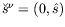is the polarization unit vector in the rest frame and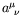is a Lorentz transformation from the rest frame. Thus we also have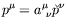, where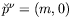. This tells us that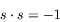(5.201)

and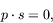(5.202)

which is true in any frame since they are Lorentz scalars. Thus in the rest frame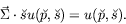(5.203)

Let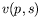denote a spinor of negative energy, with polarization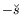in the reset frame. In this case,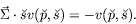(5.204)

Therefore in an arbitrary Lorentz frame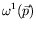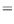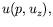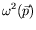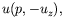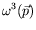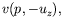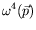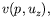(5.205)

where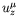is a 4-vector, which in the rest frame is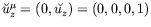. An arbitrary spinor is thus specified by the sign of the energy, its momentum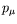, and polarization in the rest frame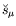.Next: Projection Operators for Energy Up: Plane-Wave Solutions Previous: Plane-Wave Solutions
Douglas M. Gingrich (gingrich@ ualberta.ca)
2004-03-18